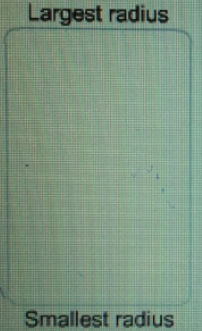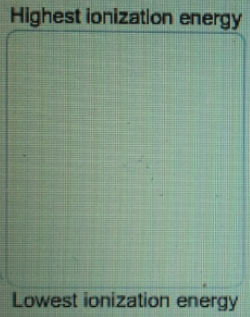# Problem: Rank these atoms and ions according to radius.K-        K+       KRank these ions according to ionic radius.Sr2+        As3-      Se2-      Br-      Rb+ Rank these elements according to first ionization energy. Sb      N       As      P

###### FREE Expert Solution

We’re being asked to Rank these atoms and ions according to radius.

Recall that ionic radius is the size of an ion. The trend for ionic radius is as follows: it increases from right to left and down a period in the periodic table. Ionic radius also increases with increasing number of electrons.

K-, K and K

• Since the element is the same, the charge could easily tell us the size.
• more negative the charge→ more electrons → larger radius

Ranking from largest to smallest radius:

K> K > K+

Sr2+ ,As3-,Se2-,Brand Rb

• All the ions are isoelectronic with Kr (same number of electrons).

Because of this, the ionic radius will just depend on the charge of the ion: the more positive the charge of an ion is, the smaller the ion is.

–3 > –2 > –1 > 0 > +1 > +2 > +3

Ranking from largest to smallest radius:

As3- > Se2-Br> RbSr2+###### Problem Details

Rank these atoms and ions according to radius.K-        K+       K

Rank these ions according to ionic radius.Sr2+        As3-      Se2-      Br     Rb

Rank these elements according to first ionization energy.Sb      N       As      P